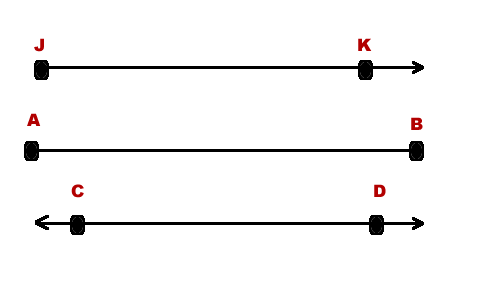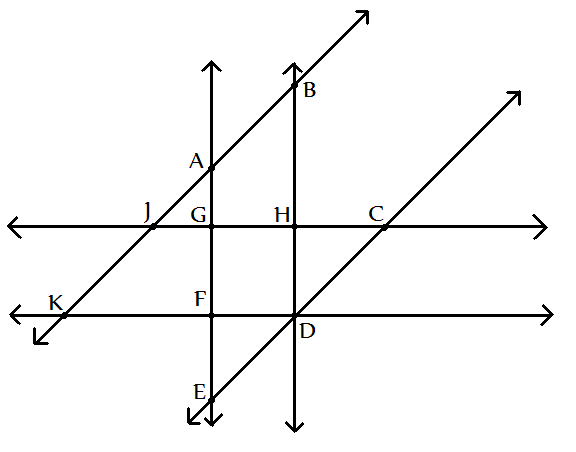# Difference between Line Segment and Ray

Line segments and rays are different mathematical representations that are used in various subjects, such as physics, astronomy, geometry etc.

Geometry is one of the basic subjects in math and the tenets of this subject are the concepts of lines and rays. Lines and line segments are the same things and the main difference between them and rays, is that the latters originate from a specific place where as the lines are mostly used as connective agents between two things or points. Lines do not contain arrows at the end where as in the concept of rays, the arrows are necessary as they point towards the direction of movement.

Another difference is that there is no limit to the number of rays originating from a specific point, whereas this not the case in line segments, where the line only travels between two points.

Lines and rays may have different implications in various subjects and if we get out of the field of math, and enter the prospective studies of physics then rays and line segments may have different meanings.

Consider the examples of scalars and vectors. The scalars are represented by line segments, which indicate the magnitude of any parametre like speed, displacement etc. The vectors on the other hand are represented by rays, with the line showing the magnitude of the the vector and the arrow pointing towards the direction, for eg, velocity, acceleration etc. There are further parametres like tensors which have more than one directions attached, but that will make the basic understanding of the difference at hand, a bit more complicated.

### Instructions

• 1

Line Segment

A line segment is used to bridge a connection between two points in math.

- Image Courtesy: freemathhelp.com• 2

Ray

A ray is used as a line that denotes the direction of travel in math and must contain the arrowheads for this purpose.

- Image Courtesy: freemathhelp.com•